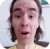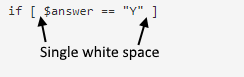FreeKB - Bash Shell Scripting if, elif, else, then statements
Bash Shell Scripting - if, elif, else, then statementsIn bash, an if statement has the following structure.

``````if [ comparison ]; then
--do something--
elif [ comparison ]
--do something
else
--do something--
fi``````

For example.

``````if [ \$foo -eq 1 ]; then
echo "Foo equals 1"

elif [ \$foo -eq 2 ]
echo "Foo equals 2"

else
echo "Foo does not equal 1 or 2"

fi``````

The following table contains commonly used comparison operators.

 Equals (integers) if [ \$foo -eq 1 ]; then Does not equal (integers) if [ \$foo -ne 1 ]; then Equals (strings) if [ "\$foo" == "Hello" ]; then Does not equal (strings) if [ "\$foo" != "Hello" ]; then Greater than if [ \$foo -gt 1 ]; then Greater than or equal to if [ \$foo -ge 1 ]; then Less than if [ \$foo -lt 1 ]; then Less than or equal to if [ \$foo -le 1 ]; then Variable contains no value (null / empty) if [ -z "\$foo" ]; then Variable contains a value (not null / not empty) if [ ! -z "\$foo" ]; then File exists if [ -e "/path/to/file" ]; then File does not exist if [ ! -e "/path/to/file" ]; then File is empty if [ ! -s "/path/to/file" ]; then File is not empty if [ -s "/path/to/file" ]; then File contains if grep --quiet "Hello World" /path/to/file; then File does not contain if ! grep --quiet "Hello World" /path/to/file; then Directory exists if [ -d "/home/test" ]; then Directory does not exist if [ ! -d "/home/test" ]; then Directory empty if [ -z "\$(ls -A /path/to/directory)" ]; then Directory not empty if [ ! -z "\$(ls -A /path/to/directory)" ]; then Variable contains if [[ \$foo = *Hello* ]]; then Variable does not contain if [[ \$foo != *Hello* ]]; then Variable begins with if [[ \$foo =~ ^Hello ]]; then Variable does not begin with if [[ ! \$foo =~ ^Hello ]]; then Variable ends with (do not place double quotes around value) if [[ \$foo =~ Hello\$ ]]; then Variable does not end with (do not place double quotes around value) if [[ ! \$foo =~ Hello\$ ]]; then Array contains if [[ "\${array[@]}" =~ "\$value" ]]; then Array does not contain if [[ ! "\${array[@]}" =~ "\$value" ]]; then

Spacing

Many if statements requre a single white space inside of the brackets. I also use a single white space as my practice.And (&&), Or (||)

Double && characters can be used to link statements together as an and.

``````if [ \$foo -eq 1 ] && [ \$bar -eq 1 ]
``````
``````if [[ \$foo == "Hello" && \$bar == "World" ]]
``````

Double || characters can be used to link statements together as an or.

``if [ \$foo -eq 1 ] || [ \$bar -eq 1 ]``
``````if [[ \$foo == "Hello" || \$bar == "World" ]]
``````

We will never share your name or email with anyone. Enter your email if you would like to be notified when we respond to your comment.

Please enter 00b98 in the box below so that we can be sure you are a human.

Web design by yours truely - me, myself, and I   |   jeremy.canfield@freekb.net   |